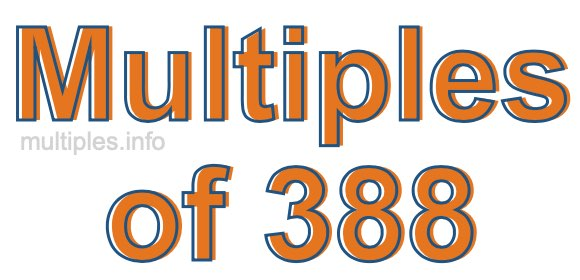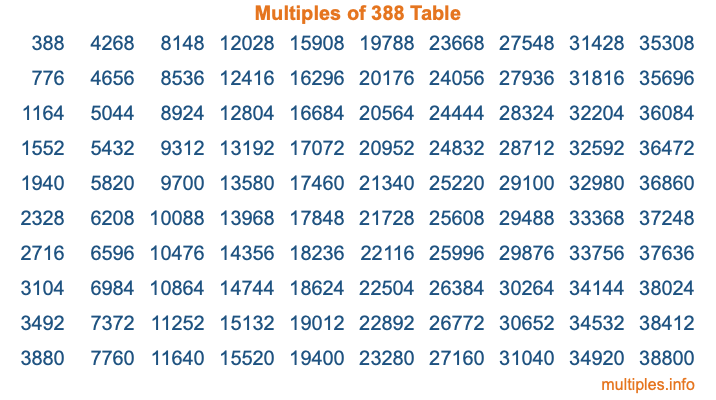Multiples of 388Welcome to the Multiples of 388 page. Here we will first teach you everything you will ever need to know about the multiples of 388, and then give you a study guide summary of everything we taught you to make sure you remember it all. Use this page to look up facts and learn information about the multiples of 388. This page will make you a multiples of three hundred eighty-eight expert!

Definition of Multiples of 388
Multiples of 388 are all the numbers that when divided by 388 equal an integer. Each of the multiples of 388 are called a multiple. A multiple of 388 is created by multiplying 388 by an integer.

Therefore, to create a list of multiples of 388, you start with 1 multiplied by 388, then 2 multiplied by 388, then 3 multiplied by 388, and so on for as long as you want. Thus, the list of the first five multiples of 388 is 388, 776, 1164, 1552, and 1940. To see a larger list of multiples of 388, see the printable image of Multiples of 388 further down on this page. We also have a category where you can choose any nth multiple of 388.

Multiples of 388 Checker
The Multiples of 388 Checker below checks to see if any number of your choice is a multiple of 388. In other words, it checks to see if there is any number (integer) that when multiplied by 388 will equal your number. To do that, we divide your number by 388. If the the quotient is an integer, then your number is a multiple of 388.

Is  a multiple of 388?

Least Common Multiple of 388 and ...
A Least Common Multiple (LCM) is the lowest multiple that two or more numbers have in common. This is also called the smallest common multiple or lowest common multiple and is useful to know when you are adding our subtracting fractions. Enter one or more numbers below (388 is already entered) to find the LCM.

Check out our LCM Calculator if you need more details about the Least Common Multiple or if you need the LCM for different numbers for adding and subtraction fractions.

nth Multiple of 388
As we stated above, 388 is the first multiple of 388, 776 is the second multiple of 388, 1164 is the third multiple of 388, and so on. Enter a number below to find the nth multiple of 388.

th multiple of 388

Multiples of 388 vs Factors of 388
388 is a multiple of 388 and a factor of 388, but that is where the similarities end. All postive multiples of 388 are 388 or greater than 388. All positive factors of 388 are 388 or less than 388.

Below is the beginning list of multiples of 388 and the factors of 388 so you can compare:

Multiples of 388: 388, 776, 1164, 1552, 1940, etc.

Factors of 388: 1, 2, 4, 97, 194, 388

As you can see, the multiples of 388 are all the numbers that you can divide by 388 to get a whole number. The factors of 388, on the other hand, are all the whole numbers that you can multiply by another whole number to get 388.

It's also interesting to note that if a number (x) is a factor of 388, then 388 will also be a multiple of that number (x).

Multiples of 388 vs Divisors of 388
The divisors of 388 are all the integers that 388 can be divided by evenly. Below is a list of the divisors of 388.

Divisors of 388: 1, 2, 4, 97, 194, 388

The interesting thing to note here is that if you take any multiple of 388 and divide it by a divisor of 388, you will see that the quotient is an integer.

Multiples of 388 Table
Below is an image of the first 100 multiples of 388 in a table. The table is in chronological order, column by column. The first column has the first ten multiples of 388, the second column has the next ten multiples of 388, and so on.The Multiples of 388 Table is also referred to as the 388 Times Table or Times Table of 388. You are welcome to print out our table for your studies.

Negative Multiples of 388
Although not often discussed or needed in math, it is worth mentioning that you can make a list of negative multiples of 388 by multiplying 388 by -1, then by -2, then by -3, and so on, to get the following list of negative multiples of 388:

-388, -776, -1164, -1552, -1940, etc.

Multiples of 388 Summary
Below is a summary of important Multiples of 388 facts that we have discussed on this page. To retain the knowledge on this page, we recommend that you read through the summary and explain to yourself or a study partner why they hold true.

There are an infinite number of multiples of 388.

A multiple of 388 divided by 388 will equal a whole number.

388 divided by a factor of 388 equals a divisor of 388.

The nth multiple of 388 is n times 388.

The largest factor of 388 is equal to the first positive multiple of 388.

388 is a multiple of every factor of 388.

388 is a multiple of 388.

A multiple of 388 divided by a divisor of 388 equals an integer.

388 divided by a divisor of 388 equals a factor of 388.

Any integer times 388 will equal a multiple of 388.

Multiples of a Number
Here you can get the multiples of another number, all with the same attention to detail as we did for multiples of 388 on this page.

Multiples of
Multiples of 389
Did you find our page about multiples of three hundred eighty-eight educational? Do you want more knowledge? Check out the multiples of the next number on our list!

Copyright  |   Privacy Policy  |   Disclaimer  |   Contact